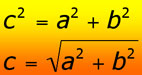# Guiding students through rearranging equations An instructor's guide to Rearranging Equations

by Dr. Jennifer M. Wenner, University of WI Oshkosh Geology, and Dr. Eric M. Baer, Highline Community College Geology.

Jump down to: Student struggles | What we left out | Resources

Many instructors find themselves struggling to get students to remember how to rearrange simple equations (like the one for velocity). There are many reasons that students need review: they may be math phobic, or have forgotten their algebra skills, or maybe they just never learned it. Nonetheless, the Manipulating Equations unit is designed to help your students catch up so that they can remember how to rearrange any equation.

This module is designed to address faculty concern that many students do not know how to manipulate equations to solve for another variable. By the time they finish this page, students should know:
1. the steps essential to manipulating equations,
2. that manipulating equations before plugging in numbers is easier (and more powerful) than inserting numbers immediately,
3. rules for manipulating equations (i.e., performing the same operation on both sides of an equation).
The manipulating equations page should help students gain a bit more confidence in their abilities to use math to solve problems that are common in introductory geoscience.

## Why is it hard for students?

Students struggle with equation manipulation for a variety of reasons:1. As with many topics covered on this website, one obstacle to learning how to manipulate equations is math phobia - many students believe that they can't "do math". This belief is so strong that they generate a mental block and are often unwilling to even consider trying to manipulate an equation. Giving students support in building their math skills may help some of them to overcome this problem.
2. Many students have the impression that there is "magic" in numbers. Thus, they immediately plug in numbers and try to rearrange the equation with the numbers. Sometimes this works but often, they cannot see their way to isolating the unknown variable when there are numbers in the way. This can also lead to problems with units if they don't keep track of what units go with what number!
3. In addition to the "magic numbers" problem, some students are intimidated by the idea of manipulating equations with only "variables" in them. This may be because they feel more comfortable performing an operation on a number (e.g., multiplying by by 8 or 42) than on a letter (m or v). This is compounded even further if there is a Greek letter like ρ or θ involved in the equation.
4. Students think they should just be able to use the formula that they memorized (e.g., rate = distance/time) to solve all problems in a single step. This page (and the associated practice problems) attempts to get them to do more than one step.

This module is designed to remind students of the simple mathematicsthat they may have forgotten. We only address simple mathematical operations: addition, subtraction, multiplication and division. The rules will work for any other operations (such as exponents and other operations). When these types of operations come up in other parts of this site, they are addressed with in these pages (e.g., the trigonometry page addresses inverse trig functions).